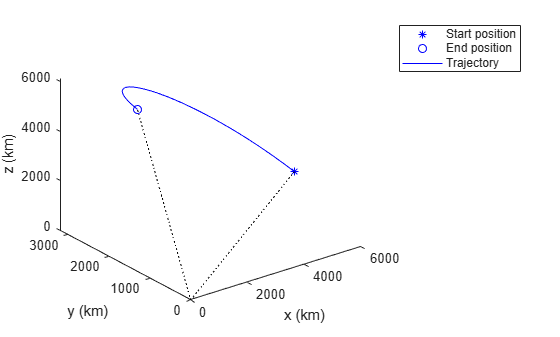# lookupPose

Obtain pose of geodetic trajectory for a certain time

Since R2018b

## Syntax

``[position,orientation,velocity,acceleration,angularVelocity,ecef2ref] = lookupPose(traj,sampleTimes)``
``[___] = lookupPose(traj,sampleTimes,coordinateSystem)``

## Description

example

````[position,orientation,velocity,acceleration,angularVelocity,ecef2ref] = lookupPose(traj,sampleTimes)` returns the pose information of the waypoint trajectory at the specified sample times. If any sample time is beyond the duration of the trajectory, the corresponding pose information is returned as `NaN`.```
````[___] = lookupPose(traj,sampleTimes,coordinateSystem)` additionally enables you to specify the format of the `position` output.```

## Examples

collapse all

Create a `geoTrajectory` with starting LLA at [15 15 0] and ending LLA at [75 75 100]. Set the flight time to ten hours. Sample the trajectory every 1000 seconds.

```startLLA = [15 15 0]; endLLA = [75 75 100]; timeOfTravel = [0 3600*10]; sampleRate = 0.001; trajectory = geoTrajectory([startLLA;endLLA],timeOfTravel,'SampleRate',sampleRate);```

Output the LLA waypoints of the trajectory.

```positionsLLA = startLLA; while ~isDone(trajectory) positionsLLA = [positionsLLA;trajectory()]; end positionsLLA```
```positionsLLA = 37×3 15.0000 15.0000 0 16.6667 16.6667 2.7778 18.3333 18.3333 5.5556 20.0000 20.0000 8.3333 21.6667 21.6667 11.1111 23.3333 23.3333 13.8889 25.0000 25.0000 16.6667 26.6667 26.6667 19.4444 28.3333 28.3333 22.2222 30.0000 30.0000 25.0000 ⋮ ```

Look up the Cartesian waypoints of the trajectory in the ECEF frame by using the `lookupPose` function.

```sampleTimes = 0:1000:3600*10; n = length(sampleTimes); positionsCart = lookupPose(trajectory,sampleTimes,'ECEF');```

Visualize the results in the ECEF frame.

```figure() km = 1000; plot3(positionsCart(1,1)/km,positionsCart(1,2)/km,positionsCart(1,3)/km, 'b*'); hold on; plot3(positionsCart(end,1)/km,positionsCart(end,2)/km,positionsCart(end,3)/km, 'bo'); plot3(positionsCart(:,1)/km,positionsCart(:,2)/km,positionsCart(:,3)/km,'b'); plot3([0 positionsCart(1,1)]/km,[0 positionsCart(1,2)]/km,[0 positionsCart(1,3)]/km,'k:'); plot3([0 positionsCart(end,1)]/km,[0 positionsCart(end,2)]/km,[0 positionsCart(end,3)]/km,'k:'); xlabel('x (km)'); ylabel('y (km)'); zlabel('z (km)'); legend('Start position','End position', 'Trajectory')```## Input Arguments

collapse all

Geodetic trajectory, specified as a `geoTrajectory` object.

Sample times in seconds, specified as an K-element vector of nonnegative scalars.

Coordinate system to report positions, specified as:

• `'LLA'` — Report positions as latitude in degrees, longitude in degrees, and altitude above the WGS84 reference ellipsoid in meters.

• `'ECEF'` — Report positions as Cartesian coordinates in the ECEF (Earth-Centered-Earth-Fixed) coordinate frame in meters.

.

## Output Arguments

collapse all

Geodetic positions in local reference coordinate system, returned as a K-by-3 matrix. K is the number of `SampleTimes`.

• When the `coordinateSystem` input is specified as `'LLA'`, the three elements in each row represent the latitude in degrees, longitude in degrees, and altitude above the WGS84 reference ellipsoid in meters of the geodetic waypoint.

• When the `coordinateSystem` input is specified as `'ECEF'`, the three elements in each row represent the Cartesian position coordinates in the ECEF (Earth-Centered-Earth-Fixed) coordinate frame in meters.

Data Types: `double`

Orientation in the local reference coordinate system, returned as a K-by-1 `quaternion` column vector or as a 3-by-3-by-K real array in which each 3-by-3 matrix is a rotation matrix.

Each quaternion or rotation matrix is a frame rotation from the local reference frame (NED or ENU) at the waypoint to the body frame of the target on the trajectory.

K is the number of `SampleTimes`.

Data Types: `double`

Velocity in the local reference coordinate system in meters per second, returned as an M-by-3 matrix.

K is specified by the `SamplesPerFrame` property.

Data Types: `double`

Acceleration in the local reference coordinate system in meters per second squared, returned as an M-by-3 matrix.

K is the number of `SampleTimes`.

Data Types: `double`

Angular velocity in the local reference coordinate system in radians per second, returned as a K-by-3 matrix.

K is the number of `SampleTimes`.

Data Types: `double`

Orientation of the reference frame with respect to the ECEF (Earth-Centered-Earth-Fixed) frame, returned as a K-by-1 `quaternion` column vector or as a 3-by-3-by-K real array, in which each 3-by-3 matrix is a rotation matrix.

Each quaternion or 3-by-3 rotation matrix is a frame rotation from the ECEF frame to the local reference frame (NED or ENU) at the current trajectory position.

K is the number of `SampleTimes`.

Data Types: `double`

## Version History

Introduced in R2018b